﻿ Numerical Study of the Flow Upstream of a Water Intake Hydroelectric Dam in Stationary RegimePublications are Open
Access in this journal
Article Versions
Export Article
• Normal Style
• MLA Style
• APA Style
• Chicago Style
Research Article
Open Access Peer-reviewed

### Numerical Study of the Flow Upstream of a Water Intake Hydroelectric Dam in Stationary Regime

Tchawe Tchawe Moukam , Djiako Thomas, Kenmeugne Bienvenu, Tcheukam-Toko Denis
American Journal of Energy Research. 2018, 6(2), 35-41. DOI: 10.12691/ajer-6-2-1
Received July 01, 2018; Revised August 16, 2018; Accepted August 29, 2018

### Abstract

The use of hydraulic power in the field of electricity generation has long been a majority, because it begins in antiquity by so-called traditional means. In this work, it is proposed to study the variation of the flow upstream of the intake of a penstock, at different Reynolds numbers. The objective is to understand the influence of the step upstream and the intake, on the structure of the flow in this zone. This study is conducted by a numerical approach under FLUENT (6.3.26) in 2D. There is an interesting variation of the velocity in the studied area. From this section comes out two zones classified as follows: a zone of elevated flow velocities towards the center of the dam (natural center of the intake), and a zone of diminishing of this one which is on both sides of the center of the dam and facing obstacles. Similarly upstream of the step, a recirculation zone is observed; its presence is clearly significant on the structure of the flow at the position x = 9m. We are just above the step at the beginning of a change in the flow regime.

### 1. Introduction

World production of hydropower-based electricity is about 20% of total electricity production 1. This production is still dominated by fossil fuels, which still hold about 60% of the world's electricity production 2.

A dam is erected at a strangulation of the banks of a watercourse; which creates a reservoir of water. Turbines are supplied with water under pressure through a system of pipelines and flow regulators 3. This supply under pressure is carried out by the penstock. According to Achour B. 4, it is that of gravity driving supplying, for energy needs, a hydroelectric plant.

The purpose of this study is to understand the behavior of the flow upstream of a specific dam, and the impact of its orifice on the structure of the flow. Some studies have been conducted in this direction; this is the case of Shamman et al. 5 where Potential flow solutions of a point/line sink are extended to study the velocity field upstream of a finite-size orifice and sluice gate. The results obtained numerically and experimentally compare very well. In the same logic, Bryant et al. 6 utilise a physical model study to investigate the flow field upstream of orifices. In particular, new experimental data for the upstream flow pattern resulting from multiple orifices, an orifice near a free surface, and a large orifice (where the pressure gradient across the orifice cannot be ignored) are collected and presented. In addition to the proposed solution that proves good with measurement data, the skew in the velocity profile in the vertical direction due to the pressure gradient is accurately predicted.

Islam et al. 7 shows that the Schwarz-Christoffel transformation can be used to estimate the flow upstream of two-dimensional rectangular intakes having variable sizes and locations and for nozzle-shaped intakes. Like previous studies, digital and experimental studies fit well together. Note that many other studies have been conducted in the same field.

In addition to the previous studies, work on the forward-facing step is required to gain a deeper understanding of upstream flow behavior. This is the case of studies of Abdalla et al. 8 who study the flow structure of a transitional separated-reattached flow over a surface mounted obstacle and a forward-facing step. To do this, the Large-eddy simulation (LES) of transitional separating-reattaching flow on a two-dimensional square surface-mounted obstacle and a forward facing step has been performed using a dynamic sub-grid scale model. The transition process has been elucidated using both 2D and 3D flow visualisation of the flow. Karathanassis I. K. et al. 9 searched for the flow effects on forced convection heat transfer in a channel with stepwise-varying width.

Other older studies may be mentioned such as those of Moss and Baker 10, Barbosa et al. 11 to name only these.

This work focuses on the upstream part of the Song-loulou dam, with a non-focused look on the intake that is the subject of another study. The variation of the Reynolds number between the maximum flow and the minimum permissible flow (for a maximum power) will allow us to show the velocity profiles at different positions of x.

Since this study is one of the first on this structure, we did a numerical study under the software Fluent 6.3.36 after modeling under Gambit 2.4.6. Then, similar studies allowed us to validate our result.

### 2. Material and Methods

The material studied here is the Songloulou dam mentioned above, which is the largest supplier of electricity in Cameroon at present. The study area is illustrated on the diagram of the figure below.

• Figure 1. Upstream area + mesh water intake

The interrupted lines represent the different positions of x in meters (x varying from 1 to 2 m). The upstream zone considered goes from x = 0m to x = 13m.

The k-ε RNG model is used for calculation. For the convective and the diffusive terms, a second order upwind method was used while the SIMPLE (Semi Implicit Method for Pressure Linked Equations) procedure was introduced for the velocity-pressure coupled to a multiphase model (VOF) 12. For the rest, Fluent's standard parameters are retained. In order to better refine results, the convergence criterion has been set at 10-8.

Simplifying Hypotheses

Given the complexity of the problem, we will impose some assumptions for our work:

• The flow is stationary;

• The fluid is incompressible;

• The turbulence is isotropic;

• The resistance of the air is negligible;

• We neglect parietal effects (work done in 2D).

General equation

Monophasic turbulent flows in a closed pipe are described by the Navier-Stokes equations. To these equations, we add the equation of turbulent kinetic energy and that of its dissipation as proposed by Launder and Spalding 13.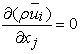(1)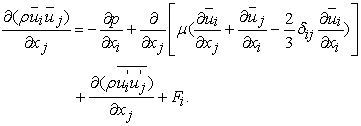(2)

where (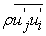) is the Reynolds tensor.

Relationship (1) is the continuity equation, and (2) the quantity of motion conservation equation with:

• The left term represents convective transport;

• The first term on the right represents the forces due to pressure;

• The second term on the right represents the viscosity forces;

• The last two terms on the right represent the forces generated by the turbulence.

The closure problem is solved using the Boussinesq hypothesis: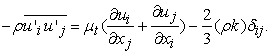(3)

Dimensional analysis provides a scale value of large vortices and allows the viscosity to be related to the two variables k and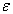. We show a coefficient of proportionality that is obtained experimentally: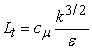(4)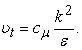(5)

To determine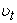, it is necessary to calculate the two variables k and. The equations of turbulent kinetic energy and its dissipation rate give us: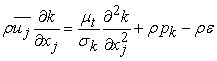(6)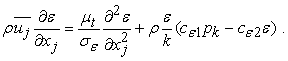(7)

These equations can be rewritten for turbulent kinetic energy in the form: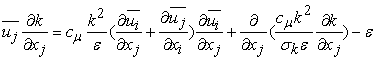(8)

• The term on the left represents the variation of turbulent kinetic energy;

• The first term on the right represents the production of turbulent kinetic energy;

• The second term on the right represents diffusion;

• The last term of the right, the dissipation.

The equation of dissipation energy is given by the equation: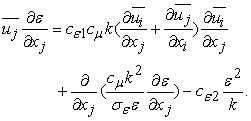(9)

Constants determined from elementary experiences are: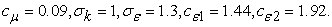(10)

### 3. Results and Discussion

Boundary condition

The water level of the dam is that of the period of the low level for maximum power (low water for maximum flow). The flow rate therefore varies from 130m3/s to 152m3/s.

Velocity profiles at different positions.

At this level of the upstream bed, the flow is substantially stationary as shown by the velocity profiles above. We are just noticing a steady increase in flow velocity with increasing Reynolds number. The study Islam et al. 7, Marghzar et al. 14 provide similar results.

From this position, variations are observed on the flow structure. Like Figure 2 (a), the variation of the velocity remains constant with the Reynolds number.

The variation in the flow structure is more and more observed. In Figure 2 (c), the change in flow velocity is no longer completely constant at all water levels in the upstream reservoir. It is more pronounced in Figure 2 (d), which shows a real decrease in the velocity of flow near the water bed approaching the forward-facing step. This is not the case for surface flow because, in the same way that the central flow uniformly expands with the variation of the Reynolds number, the flow near the free surface is rather uniformly decreasing.

Near the forward-facing step and at the point x corresponding to the location of the upstream face of the step, the effects on the structure of the flow are perceptible in the figures 2(e) and 2(f).

Indeed, the structure of the dam is made in such a way that the flow will all have to first cross a step before starting the water intake as shown in Figure 1. In Figure 2 (e), we observe a significant decrease in flow near the step at this precise position. The increase in flow velocity towards the water intake can be linearly dependent. This is not necessarily the case for Figure 2 (f), where we observe the complete cancellation of the flow velocity at the upstream step; followed by a peak of velocity just above the step. This particular case is justified by the shape of this intake which plays a funnel role to this position. Beyond the work of Meselhe et al. 15 which give similar elements, we can in the same sense state the work of Shammaa et al. 5.

We observe in Figure 2(g) the appearance of a disorder in the flow (zone enclosed in red). The peak of velocity is always maintained near the lower wall when approaching the catch; it is the confirmation of the funnel effect due to the shape of this part.

• Figure 2. Velocity profile for different Re(x106) à : (a) x=1m, (b) x=3m, (c) x=5m, (d) x=7m, (e) x=9m, (f) x=10m, (g) x=11m, (h) x=13m

Like Figure 2 (g), the disorder is not only always present, but it is even more visible. The structure of the flow is completely modified. In a two-dimensional plane, the flow goes in one direction as well as the other. This aspect is also observed in the work of Meselhe et al. 15. The return effect is characterized here by the phenomenon of recirculation, which creates its home at this precise position. This is clearly justified by the shape of the structure at this level. The observed angular deviation (slope) creates an abyss in which water stagnates; depending on the velocity of the flow, the recirculation is either filled or attenuated. We can say according to our results that not only is the birthplace of the recirculation phenomenon observed above known, but also the place of maximum recirculation. Likewise, the lower the Reynolds number, the higher the recirculation phenomenon at this level. Its direct consequence on our structure and in the penstock is the birth of the air bubbles; which will contribute to the phenomenon of cavitation on the blades of our turbine.

We agree that, within our structure, the flow regime is a function of the selected flow point (position): the farther we go, the regime becomes unsteady; on the other hand, going back to the surface, the flow regime is practically stationary.

Velocity profiles at different position of x for Re=27.52 x 106

To better visualize the variation of the flow structure at the approach of the dam (approaching the upstream step of the intake in the case of our dam), we represent below the velocity profiles at different upstream positions. . In this case, 5 positions were considered: x=1m (line1), x=3m (line2), x=5m (line3), x=7m (line4), x=9m (line5) ; knowing that the upstream step is at the position x=10m of the considered zero point.

• Figure 3. Velocity profile at differentes position (Re=27.52x106)

By imposing the average flow upstream, we notice an increasing slowing of the flow upstream of the step near the water bed and this, on a significant height (slightly proportional to the height of the threshold). After the position x=7m, there is a rapid decrease in the flow velocity and x=9m, there is practically no cancellation of the velocity at this level.

On the other hand, towards the axis of the structure (part which leads to the pipe), one observes an increasing acceleration of the velocity of water; which makes us conclude that this part is the place “par excellence” of the transformation of the flow regime.

### 4. Comparative Study

The work of Islam et al. 7 accompanied by those of Roth and Hager 16 comfort ours upstream of the orifice and therefore are used to validate our results in this part of the dam. Similarly, those of Abdalla et al. 8 accompanied by Moss and Baker 10, and those of Karathanassis et al. 9 accompanied by those of Barbosa et al. 11 will help us on the effects of the step.

En amont du seuil

• Figure 4. Velocity profile approaching the orifice for: (a) x=1m, (b) x=3m, (c) x=5m et (d) x=7m.

The actual behavior of the flow structure is very far from the theoretical one as we see in these different figures. The presence of the orifice is felt at a distance upstream depending on the flow rate, the diameter of the orifice and also the shape of the orifice.

However, using a certain number of studies carried out upstream of an orifice, we can conclude that whatever the configuration of the system to study, the structure of the flow makes appear a high velocity towards the natural axis of the orifice.

Sur le seuil (après la face amont)

• Figure 5. Velocity profile on the step at x=11m

The structure clearly shows the recirculation phenomenon close to the step structure, where negative velocity is observed. Unlike the other works considered that were completely realized with free surface, ours has the peculiarity that the flow on the step is directed towards a convergent which characterizes the intake of water of our pipe; hence justifying the shape and peak of the profile. Overall, we notice that the flow is more accelerated not far from the step structure.

### 5. Conclusion

The shape of the dam near the intake has a huge impact on the structure of our upstream flow. The incoming flow is assumed to be stationary with a constant variation in the flow structure as a function of the Reynolds number. However, the presence of the forward-facing step modifies it so that, the closer one gets to the step, the more the flow velocity decreases near the water bed; it is higher over a certain height H corresponding approximately to the size of the step. A recirculation zone is observed upstream. It has the particularity of generating disorder in the structure of the flow.

On the step, the structure of the flow is completely changed. The flow goes in one direction as in the other depending on the chosen point. The peak velocity is observed not far from the step but the shape of the flow structure is more curved than that used for comparison. This is due not only to the presence of an orifice downstream of the step, but also to the shape of the water intake orifice.

Depending on the different geometries in the framework of the structure upstream of the intake, the incoming flow is completely modified. We move from a stationary regime to an unsteady state.

### References

  Mohammed T. G. and Rasim A. K, Hydro Power, 31 p. In article  Ketcheuzeu-Ngakam J., Koueni-Toko C., Djeumako B., Tcheukam-Toko D., Kuitche A, Characterization of hydrodynamics parameters of a Kaplan turbine, Energy and Power (EP), Vol. 4, issue 3, 2014, pp. 58-69. In article  Energy of Cameroon S.A. In article  Achour B, Conduite et canaux en charge : avant-propos, 2007, 4P. In article  Yaser Shammaa, David Z. Zhu, and Nallamuthu Rajaratnam, Flow Upstream of Orifices and Sluice Gates. Journal of Hydraulic Engineering, Vol. 131, No. 2, February 2005. In article View Article  Bryant D. B., Khan A. A., and Aziz N. M. Investigation of flow Upstream of Orifices, Journal of Hydraulic Engineering, Vol. 134, No. 1, January 2008. In article View Article  Md Rashedul Islam and David Z. Zhu, M. Flow Upstream of Two-Dimensional Intakes, Journal of Hydraulic Engineering, Vol. 137, No. 1 2011. In article  Ibrahim Elrayah Abdalla, Zhiyin Yang and Malcolm Cook. Computational analysis and flow structure of a transitional separated-reattached flow over a surface mounted obstacle and a forward-facing step, International Journal of Computational Fluid Dynamics, Vol. 23, No. 1 2009. 25-57 In article View Article  Karathanassis I.K., Papanicolaou E., Belessiotis V., Bergeles G.C. Three-dimensional flow effects on forced convection heat transfer in a channel with stepwise-varying width, International Journal of Thermal Sciences 67, 2013,177-191. In article View Article  Moss, W.D. and Baker, S. Re-circulating Flows Associated with Two-dimensional Steps, Aeronautics Quarterly, 1980,151-172. In article View Article  Barbosa Saldana J.G., Quinto Diez P., Sanchez Silva F. and Carvajal Mariscal I. Numerical simulation for the flow structures following a three-dimensional horizontal forward-facing step channel. REVISTA MEXICANA DE FISICA 53 (2) 87-95, 2007. In article  Patankar S.V. Numerical heat transfer and fluid flow. McGraw Hill, New-York. 1980. In article  Launder B. E. and Spalding D. The numerical computation of turbulent flow computational methods. Applied. Mechanical Engineering, Vol. 3, 1974, pp. 269-289. In article View Article  Sh Marghzar H., Montazerin N. and Rahimzade H. Flow field, turbulence and critical condition at a horizontal water intake, Proc. Inst. Mech. Eng., Vol. 217 Part A: J. Power and Energy, 2003, 53-62. In article  Meselhe E.A.and A.J.Odgaard. 3D numerical flow model for fish diversion studies at wanapumdam. J. Hydraul. Eng., 1998, 124:1203-1214. In article View Article  Roth, A., and Hager, W. H. Underflow of standard sluice gate, Exp. Fluids, 27, 1999, 339-350. In article View Article  Tchawe Tchawe M., Djeumako B., Koueni-Toko C., Tcheukam-Toko D. and Kuitche A. Study of Dynamic Field around a Vertical Circular Cylinder placed in an Open-Chanel Flow. International Journal of Innovative Science, Engineering & Technology, Vol. 2 Issue 2, February 2015. In article  Wilson D. Turbulence modeling for CFD. DCV industries, 2nd Edition, 1998. In article  Tcheukam-Toko D., Mokem-Chetchueng M., Mouangué R., Beda T., Murzyn F. Characterization of Hydraulic Jump over an Obstacle in Open-channel Flow. International Journal of Hydraulic Engineering (IJHE), 2(5), 2013, 71-84. In article  Fluent (2006). User manual 6.3.26. In article

Published with license by Science and Education Publishing, Copyright © 2018 Tchawe Tchawe Moukam, Djiako Thomas, Kenmeugne Bienvenu and Tcheukam-Toko DenisThis work is licensed under a Creative Commons Attribution 4.0 International License. To view a copy of this license, visit http://creativecommons.org/licenses/by/4.0/